Next: Equation of state'' of Up: Infinite Nuclear Matter Previous: Degrees of freedom

## Fermi surfaces and kinetic densities

For INM arbitrary asymmetry, the Fermi energy of each particle species is different. Finite spin densities si break the isotropy of INM, creating the possibility that the Fermi surface will deform. We are mainly interested in INM with small polarization, so we use the approximation that all Fermi surfaces are spherical.

In the mean-field approximation,
, the density of particles in momentum space with the isospin projection q and spin projection
, is
(36)

In asymmetric polarized INM, the relation between the Fermi momenta and the isoscalar scalar density reads
(37)

with
. Here,
is the average'' Fermi momentum of the whole system. The kinetic density in momentum space for each particle species is given by
(38)

and the kinetic density in coordinate space is
(39)

where
. Various kinetic densities in the spin-isospin space are given by
=
=
T0 =
T1 =
(40)

where Fm(0),
,
, and
are functions of the relative excesses:
 Fm(0) =
This is a straightforward generalization of the corresponding definition for asymmetric unpolarized nuclear matter given in . Similarly one defines
=
=
=
For calculations of INM properties, we also need derivatives of these functions. The first derivatives are given by
=
=
=
=
(41)

while the second derivatives are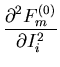=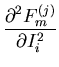=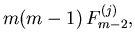(42)

for any
. Functions of the order m=0 and m=1 are rather simple:
 F0(0) =
F1(0) =
(43)

for any
. Some special values
appearing in limiting cases of INM are
 Fm(0)(0,0,0) =
Fm(0)(1,0,0) = Fm(0)(0,1,0) = Fm(0)(0,0,1) = 2m-1
=
Fm(0)(1,1,1) =
(44)

while Fm(i) = 0 if Ii = 0 and one of the other Ij's is equal to 1, with the last equal to zero. These functions are useful when writing down the equation of state and its derivatives.Next: Equation of state'' of Up: Infinite Nuclear Matter Previous: Degrees of freedom
Jacek Dobaczewski
2002-03-15